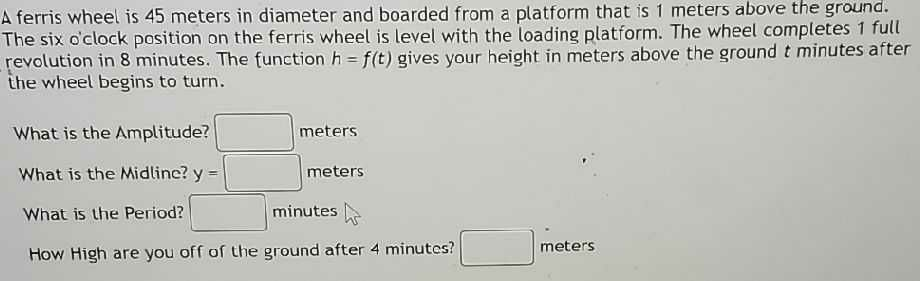### ¿Todavía tienes preguntas de matemáticas?

Pregunte a nuestros tutores expertos
Algebra
PreguntaA ferris wheel is $$45$$ meters in diameter and boarded from a platform that is $$1$$ metels above the ground. The six o'clock position on the ferris wheel is level with the loading platform. The wheel completes $$1$$ full revolution in $$8$$ minutes. The function $$h = f ( t )$$ gives your height in meters above the ground $$t$$ minutes after the wheel begins to turn.

What is the Amplitude? ()meters

What is the Midline? $$y = \square$$ meters

What is the Period? ()minutes

How High are you off of the ground after $$4$$ minutes? ()meters

$$45\\1\\8\\45$$